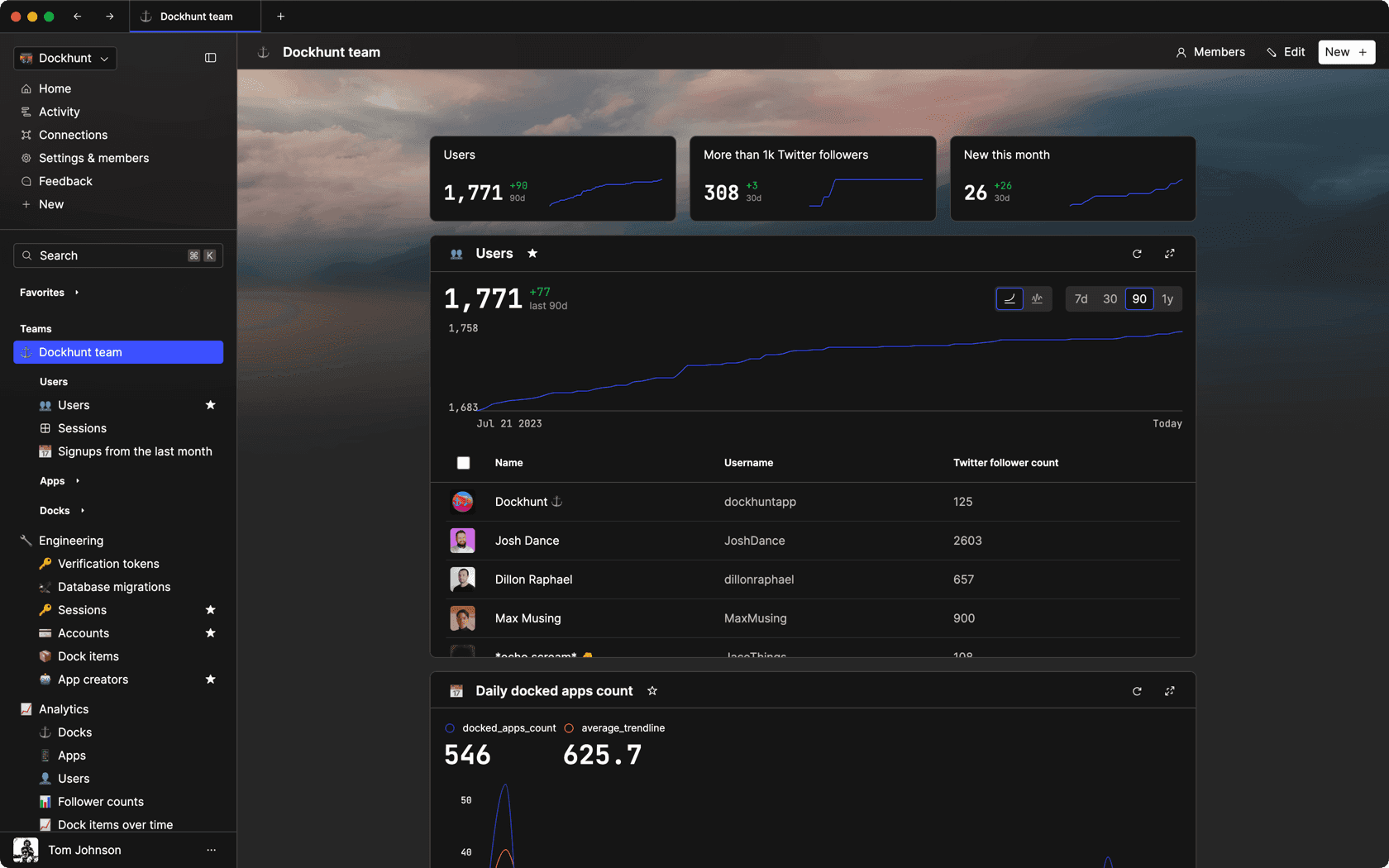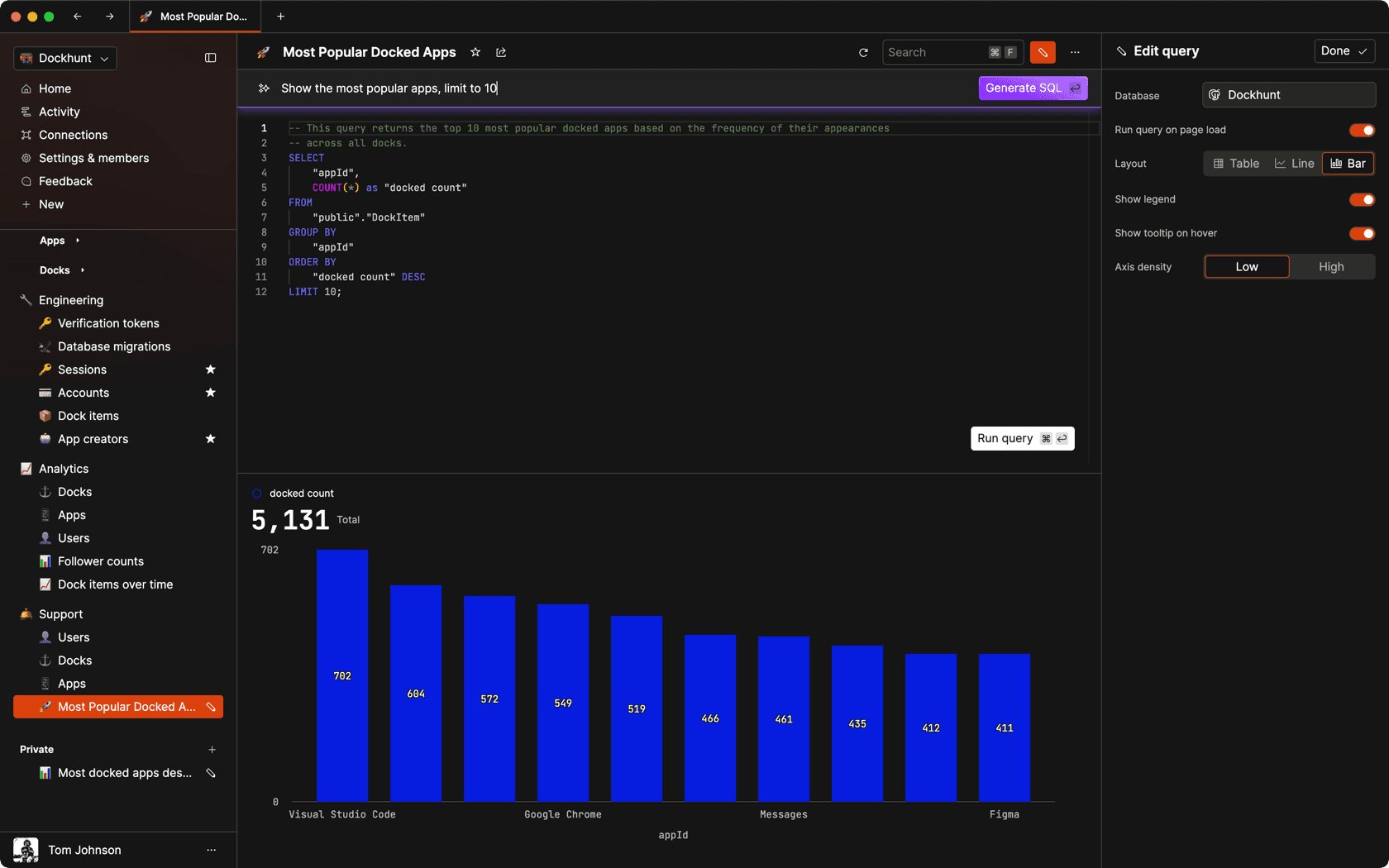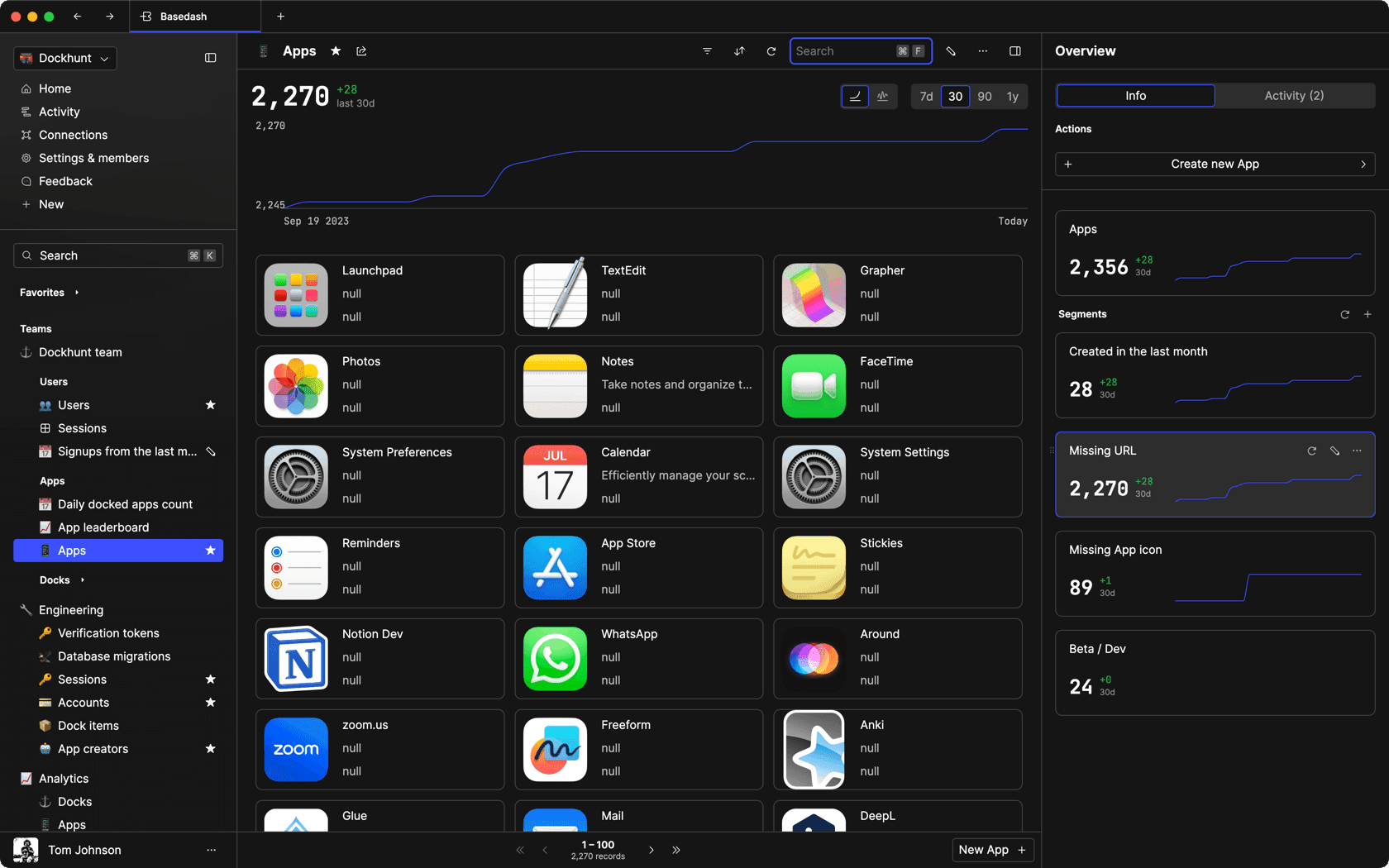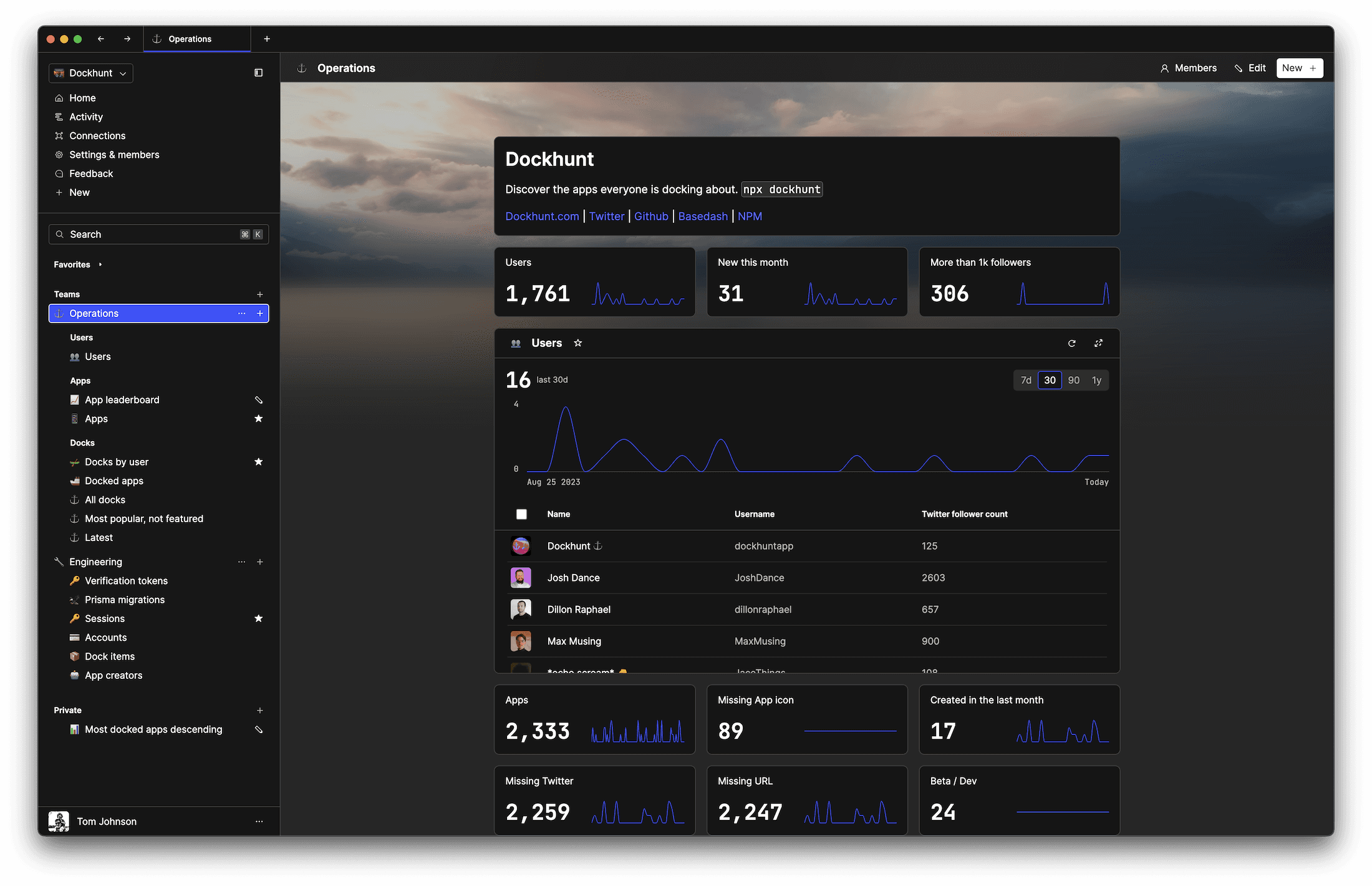# How to Reverse a Number in JavaScriptself.__wrap_n=self.__wrap_n||(self.CSS&&CSS.supports("text-wrap","balance")?1:2);self.__wrap_b=(e,r,t)=>{t=t||document.querySelector(`[data-br="\${e}"]`);let n=t.parentElement,a=s=>t.style.maxWidth=s+"px";t.style.maxWidth="";let o,i=n.clientWidth,l=n.clientHeight,c=i/2-.25,f=i+.5;if(i){for(a(c),c=Math.max(t.scrollWidth,c);c+1<f;)o=Math.round((c+f)/2),a(o),n.clientHeight===l?f=o:c=o;a(f*r+i*(1-r))}t.__wrap_o||typeof ResizeObserver<"u"&&(t.__wrap_o=new ResizeObserver(()=>{self.__wrap_b(0,+t.dataset.brr,t)})).observe(n)};self.__wrap_n!=1&&self.__wrap_b(":Rj0pm:",1)

November 8, 2023

To reverse a number in JavaScript, you need to convert the number to a string, split it into an array, reverse the array and then join it back together. This guide will walk you through the process with code snippets for clarity.

A number reversal flips the order of its digits. For example, reversing `123` yields `321`. In JavaScript, since numbers can't be directly reversed, the operation is typically performed on strings.

## Convert the number to a string

Start by converting the number to a string using the `toString()` method. This step is necessary because the reversal methods apply to strings and arrays, not numbers.

```let number = 12345;
let stringNumber = number.toString();```

## Split the string into an array

Once the number is a string, use the `split('')` method to create an array where each element is a single digit.

`let arrayDigits = stringNumber.split('');`

## Reverse the array

Use the `reverse()` method to invert the order of the elements in the array.

`let reversedArrayDigits = arrayDigits.reverse();`

## Join the array back into a string

After reversing, use `join('')` to concatenate the elements back into a single string.

`let reversedStringNumber = reversedArrayDigits.join('');`

## Convert the string back to a number

Lastly, convert the string back into a number with the `parseInt()` function. If you need to handle decimals, use `parseFloat()` instead.

`let reversedNumber = parseInt(reversedStringNumber, 10);`

## Handling negative numbers

For negative numbers, you'll need to remove the minus sign before reversing and add it back after.

```function reverseNumber(num) {
let isNegative = num < 0;
let reversedString = Math.abs(num).toString().split('').reverse().join('');
let reversedNum = parseInt(reversedString, 10);
return isNegative ? -reversedNum : reversedNum;
}```

## Dealing with decimals

If the number has decimals, the process is similar, but you'll need to handle the decimal point.

```function reverseFloat(num) {
let stringNumber = num.toString();
let parts = stringNumber.split('.');
let reversedInteger = parseInt(parts.split('').reverse().join(''), 10);
if (parts.length === 2) {
let reversedDecimal = parseInt(parts.split('').reverse().join(''), 10);
return parseFloat(`\${reversedInteger}.\${reversedDecimal}`);
}
return reversedInteger;
}```

## Final thoughts

This guide provided a method to reverse both integers and floating-point numbers. It's important to note that JavaScript may not preserve leading zeros in a reversed number since they are not significant in a numerical representation.

# Ship faster, worry less with Basedash

You're busy enough with product work to be weighed down building, maintaining, scoping and developing internal apps and admin panels. Forget all of that, and give your team the admin panel that you don't have to build. Launch in less time than it takes to run a standup.

You're busy enough with product work to be weighed down building, maintaining, scoping and developing internal apps and admin panels. Forget all of that, and give your team the admin panel that you don't have to build. Launch in less time than it takes to run a standup.

You're busy enough with product work to be weighed down building, maintaining, scoping and developing internal apps and admin panels. Forget all of that, and give your team the admin panel that you don't have to build. Launch in less time than it takes to run a standup.### Dashboards and charts

Effortlessly make charts and create a space for your team to work together towards shared goals and metrics.

### SQL composer with AISOC II
Type 2 Certified

### Features

Proudly carbon-neutral.SOC II
Type 2 Certified

### Features

Proudly carbon-neutral.SOC II
Type 2 Certified